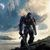Announcements
Applications are open for the 2024 Qlik Luminary Program. Apply by December 15 here.
cancel
Showing results for
Did you mean:Contributor II

## Set Analysis and Aggregation

Hi guys,

I have a peculiar problem regarding the combination of a formula that is supposed to show an aggregated median of a ratio within a statistical year.

The following formulas do work for the year that has been selected:

Median(aggr({\$<StatYear={\$(=max(StatYear))}>}sum(MeasureA/MeasureB), AggregationDimension)
Median(aggr(sum({\$<StatYear={\$(=max(StatYear))}>}MeasureA/MeasureB), AggregationDimension)

However, I want to display previous year data as a comparison, as well. So I tried the following:

Median(aggr({\$<StatYear={\$(=max(StatYear)-1)}>}sum(MeasureA/MeasureB), AggregationDimension)
Median(aggr(sum({\$<StatYear={\$(=max(StatYear)-1)}>}MeasureA/MeasureB), AggregationDimension)

But I don't receive any output, unless I select both years. I do not want to do this, though, because it would mess up the rest of my report.

Also, this applies only tor this specific formula. When simply calculating the mean or the median without any aggregation*, my report will display previous year data without any problems, even though only one year is selected.

*sum({\$<StatYear={\$(=max(StatYear)-1)}>}MeasureA/MeasureB)

*median({\$<StatYear={\$(=max(StatYear)-1)}>}MeasureA/MeasureB)

Where am I messing up?

Labels (1)
• ### General Question

2 RepliesMaster

Have you considered deriving the previous year as a variable and using that instead? Also, you might want to include the year field as part of the Aggregation Dimensions.Contributor II
Author

Hi Peter,